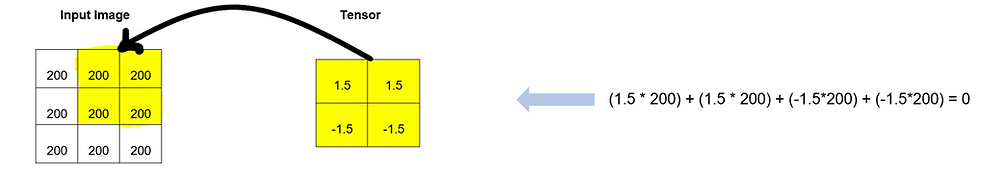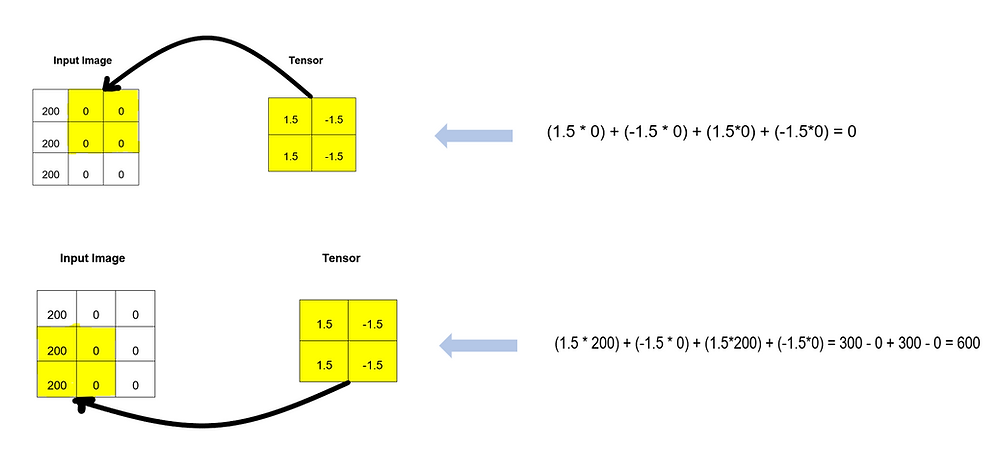Search

# Tensor / Filter: What is it simply!!

“The lens we choose, transforms the way we look at things.”

- Dewitt JonesWhen we talk about image recognition, we see Tensors in many technologies of Deep Learning. Tensors are the fundamental thing in image recognition by the machine. In this blog, I will give you a brief idea about Tensor for edge detection in image processing of Convolution Neural Network(CNN). Tensors are also called Filters.

It is very easy to understand the concept of Tensor. As we all know, older people used to read the news paper with the help of lens to magnify the text, Similarly, we use tensors in image recognition. In Layman terms, you can say it is the lens of the machine to detect the edges of the image. Edge detection is the first step in image recognition. Edges can be - horizontal or vertical in an image. Tensor runs across the entire image and it calculates the convolved numbers on each point by using a formula. Tensor is a matrix to perform operations on input image to get the convolved image. It can be size of 2X2, 3X3 or X*Y etc. Size of tensor is determined by deep learning algorithms depending on how large the data is.

Tensor for Horizontal Edge detection:

To detect the horizontal edge in an image, the numbers in the tensor should be same and transpose horizontally. For example, if tensor is 2X2 size matrix, then if top row numbers are 1.5 then both bottom numbers should be -1.5 and If -1.5 is on top row numbers then 1.5 for both bottom row numbers. It means same numbers should flip with opposite signs

horizontally. Below pictures are showing tensors for horizontal edge detection.Use of Tensor to get the Convoluted number for Horizontal Edge detection:

Tensor runs on whole image to detect the edges. Here, we will consider only a part of an input image (which is dark overall and no edge) in form of pixel matrix of size 3x3 and a tensor of 2X2 as shown below.Now, the Tensor will go on different parts of input image pixel array to calculate the convoluted numbers using the formula given below.To find the convoluted number on those pixels of input image where tensor is currently placed, we use the below formula.

(1.5 * 200) + (1.5 * 200) + (-1.5*200) + (-1.5*200) = 0

Convoluted number as a zero means there is no edge on those pixels.

Now we can calculate other Convoluted numbers for the convolved feature matrix as shown in below pictures.Now the final Convoluted Featured Matrix is shown below. All over zeros means no edge is detected in the part of the given input image.Tensor for Vertical Edge detection:

For vertical edge detection, the numbers will be flipped vertically. It means both columns will have different signs values with the same number as shown in below pictures.Use of Tensor to get the Convoluted number for Vertical Edge detection:

Suppose we have a part of an input image (which has vertical edge) in form of pixel matrix of size 3x3 and a tensor of 2X2 as shown below.Now, we will find out the convoluted numbers for above input image as we did in horizontal edge detection.To find the convoluted number on those pixels where tensor is currently placed, we will use the same formula for vertical edge detection also.

(1.5 * 200) + (-1.5 * 0) + (1.5*200) + (-1.5*0) = 300 - 0 + 300 - 0 = 600

A non-zero Convoluted number means there is an edge on those pixels.

Now we can calculate other Convoluted numbers for the Convolved feature matrix as shown in below pictures.Now the final Convoluted Featured Matrix is shown below which is showing a vertical edge in the given input image.I hope you will like this blog and will have better understanding of Tensors for edge detection in image recognition in Convolution Neural Network. I tried my best to keep it simple so that it will be easy to understand. Thank you so much for reading!!!